Question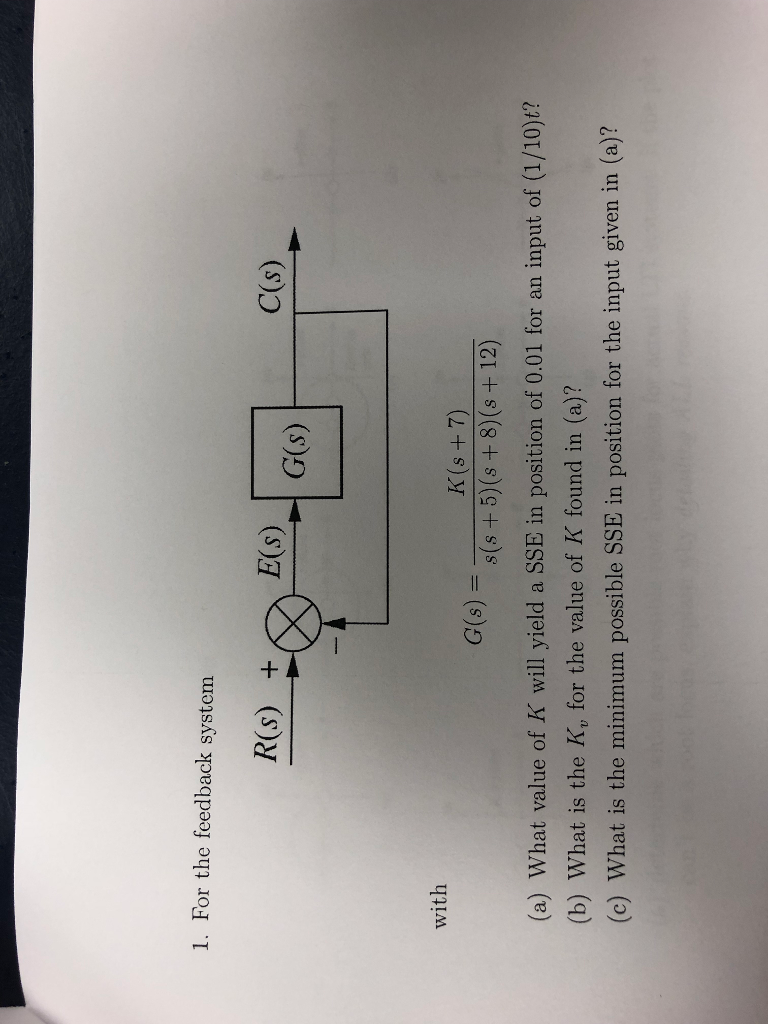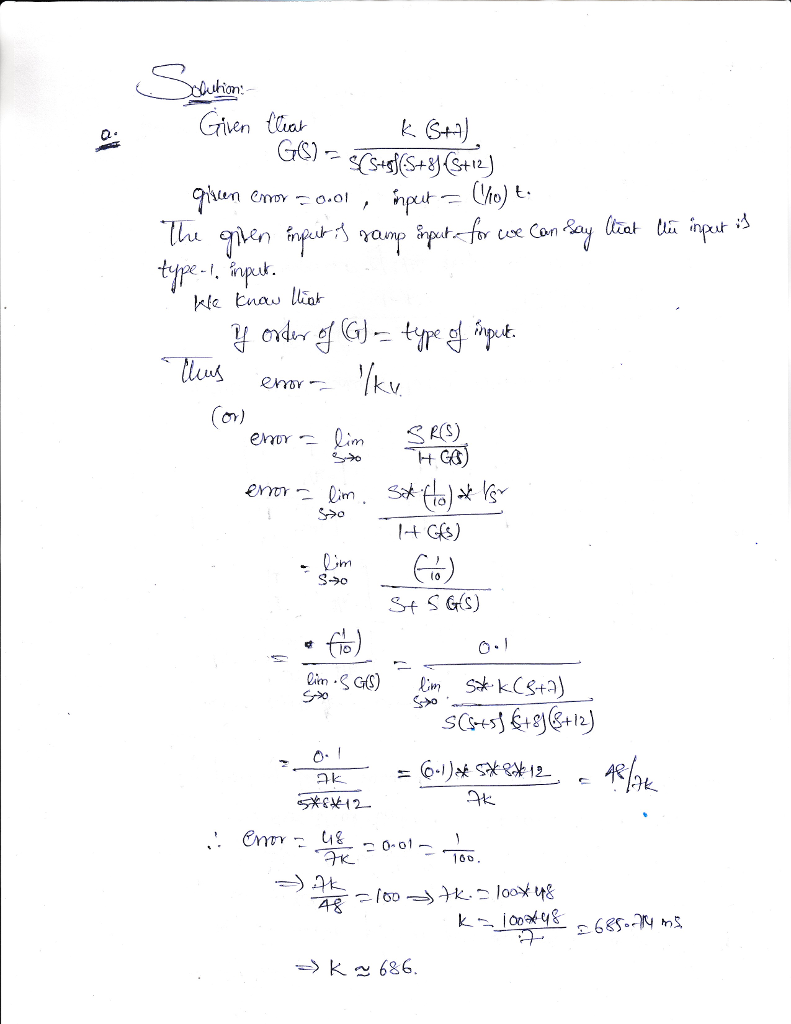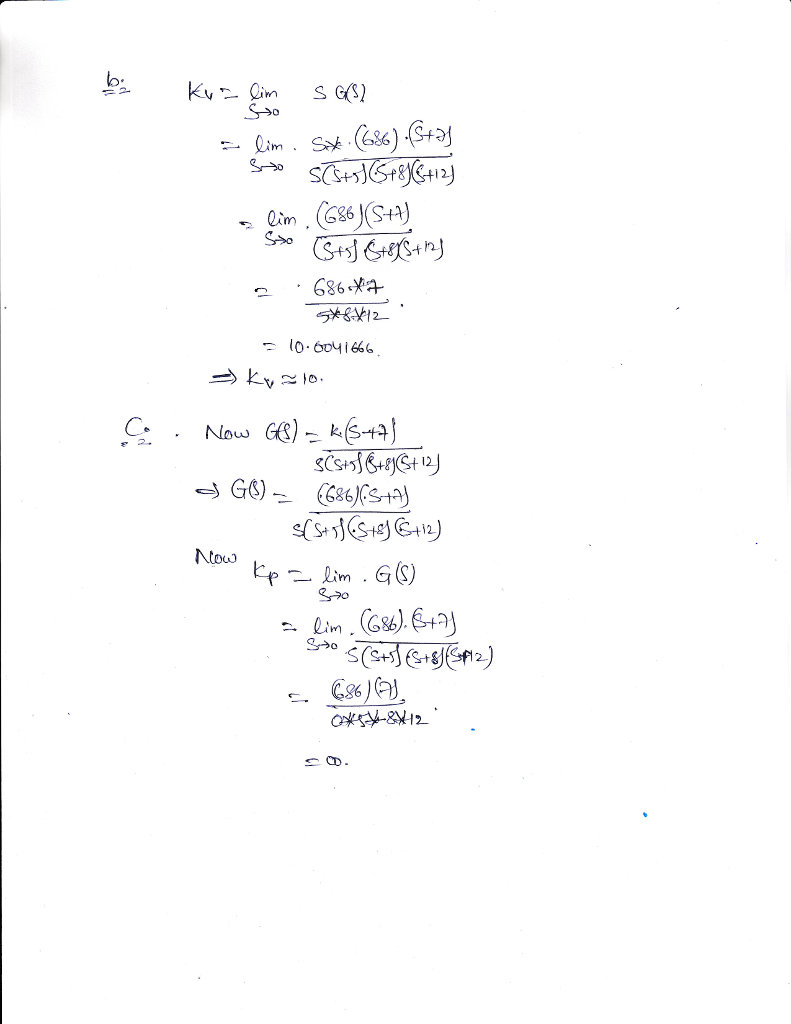#### Earn Coins

Coins can be redeemed for fabulous gifts.

Similar Homework Help Questions
• ### please answer Question 1 For the unity feedback system of figure 1.1, where Not yet answered K(s+3Xs+9) G(8) sts+6Xs...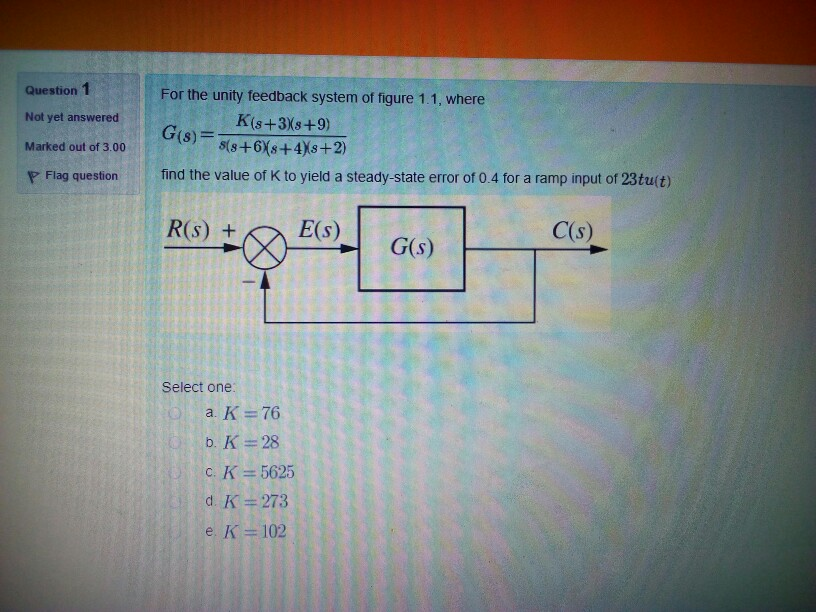please answer Question 1 For the unity feedback system of figure 1.1, where Not yet answered K(s+3Xs+9) G(8) sts+6Xs+4Xs+2) Marked out of 3.00 find the value of K to yield a steady-state error of 0.4 for a ramp input of 23tu(t) P Flag question R(s) + E(s) C(s) G(s) Select one: a. K 76 b. K 28 C. K = 5625 d. K 273 e. K 102 Question 1 For the unity feedback system of figure 1.1, where Not yet...

• ### R(s)) C(s) 1. For the unity feedback system shown above where K(s2 +2s +5) G(S)2(s +3)...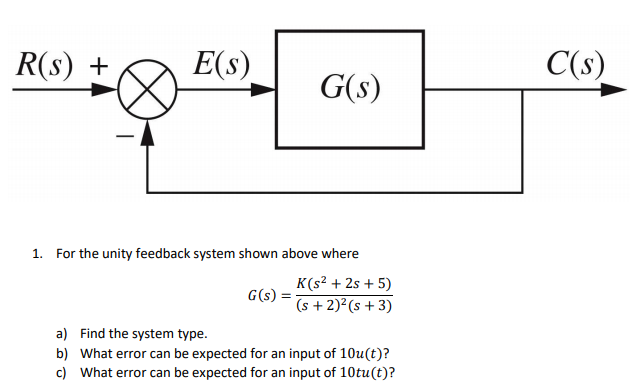R(s)) C(s) 1. For the unity feedback system shown above where K(s2 +2s +5) G(S)2(s +3) a) b) c) Find the system type. What error can be expected for an input of 10u(t)? What error can be expected for an input of 10tu(t)?

• ### 1. A unity feedback system with its forward transfer function G(s) - K(s+a)/s(s+B) is to be...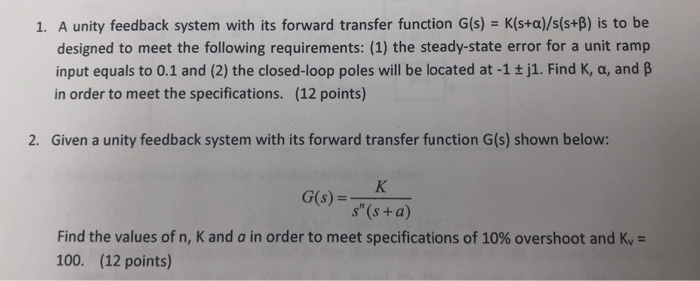1. A unity feedback system with its forward transfer function G(s) - K(s+a)/s(s+B) is to be designed to meet the following requirements: (1) the steady-state error for a unit ramp input equals to 0.1 and (2) the closed-loop poles will be located at -1 + j1. Find K, a, and B in order to meet the specifications. (12 points) 2. Given a unity feedback system with its forward transfer function G(s) shown below: s" (s +a) Find the values of...

• ### For the unity feedback system with forward loop T.F. G(s) =s(s+2)(s+4) Find, using Routh criterion, the...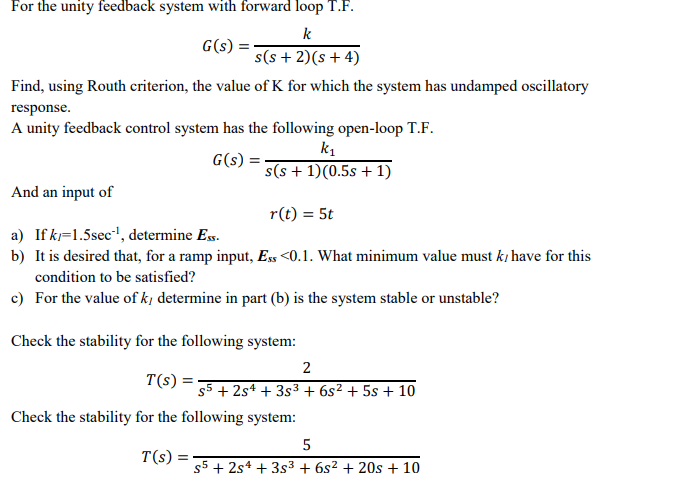For the unity feedback system with forward loop T.F. G(s) =s(s+2)(s+4) Find, using Routh criterion, the value of K for which the system has undamped oscillatory response. A unity feedback control system has the following open-loop T.F k1 s(s+1)00.5s +1) And an input of r(t) 5t b) It is desired that, for a ramp input, Ess 0.1. What minimum value must ki have for this condition to be satisfied? cFor the value of ki determine in part (b) is the...

• ### SS10. The unity-feedback system of Figure P11.1 with K (s +4) G (s) (s 2) (s 5) (s +12) is operat...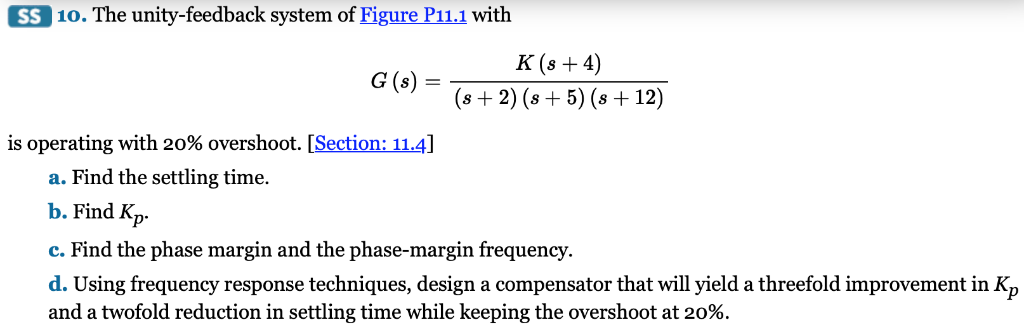SS10. The unity-feedback system of Figure P11.1 with K (s +4) G (s) (s 2) (s 5) (s +12) is operating with 20% overshoot. [Section: 114] a. Find the settling time. b. Find Kp c. Find the phase margin and the phase-margin frequency d. Using frequency response techniques, design a compensator that will yield a threefold improvement in Kp and a twofold reduction in settling time while keeping the overshoot at 20%. SS10. The unity-feedback system of Figure P11.1 with...

• ### 3. (28 pts.) The unity feedback system with K(5+3) G(s) = (s + 1)(s + 4)(s...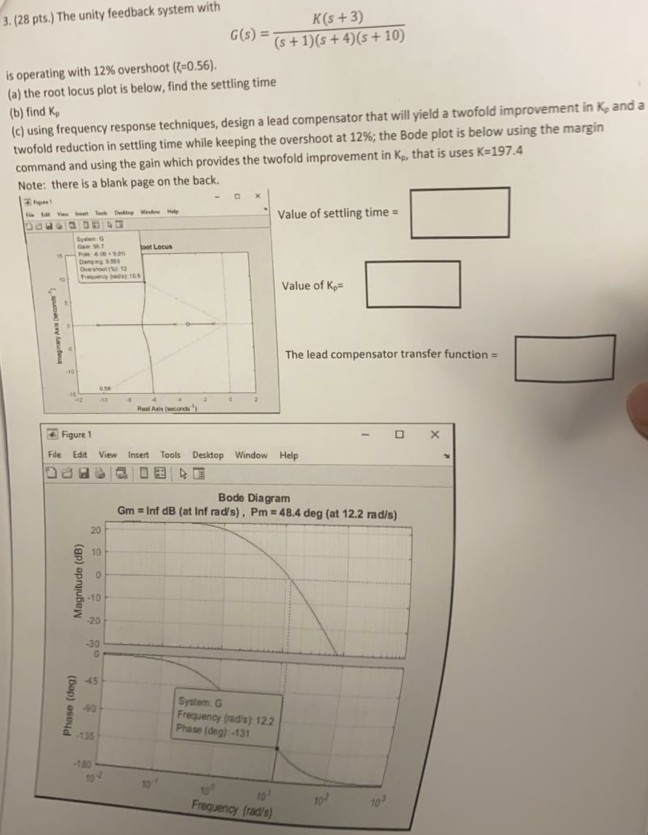3. (28 pts.) The unity feedback system with K(5+3) G(s) = (s + 1)(s + 4)(s + 10) is operating with 12% overshoot ({=0.56). (a) the root locus plot is below, find the settling time (b) find ko (c) using frequency response techniques, design a lead compensator that will yield a twofold improvement in K, and a twofold reduction in settling time while keeping the overshoot at 12%; the Bode plot is below using the margin command and using the...

• ### 1. Given the unity feedback system, where K(s+1(s 2) 1)(s-4) G(s) do the following: (a) Find the ...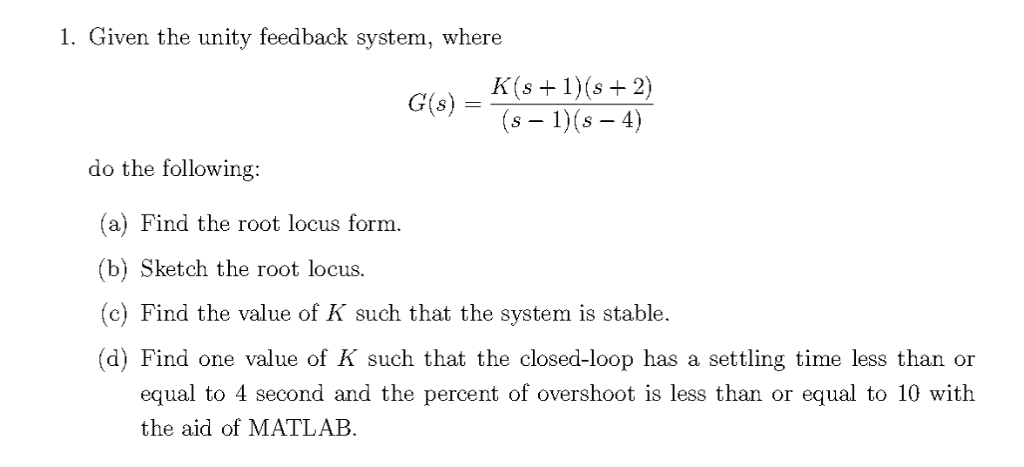1. Given the unity feedback system, where K(s+1(s 2) 1)(s-4) G(s) do the following: (a) Find the root locus form. (b) Sketch the root locus. (c) Find the value of K such that the system is stable. (d) Find one value of K such that the closed-loop has a settling time less than or equal to 4 second and the percent of overshoot is less than or equal to 10 with the aid of MATLAB 1. Given the unity feedback...

• ### Consider the unity feedback system shown below R(s) C(s) Gp(s) 5(s+6 with G,(6)(s +2)(s+25) You are...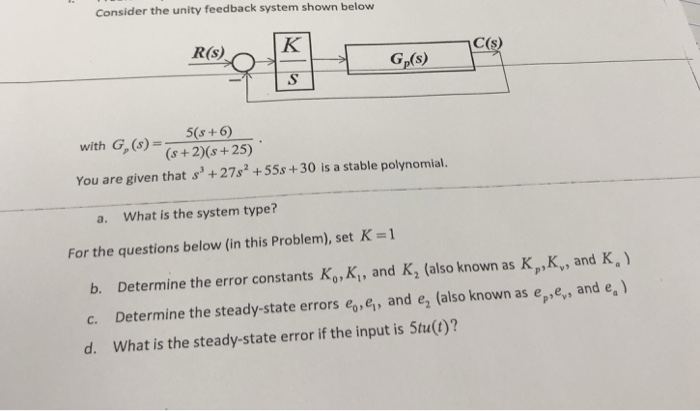Consider the unity feedback system shown below R(s) C(s) Gp(s) 5(s+6 with G,(6)(s +2)(s+25) You are given that s+27s +55s+30 is a stable polynomial. a. What is the system type? For the questions below (in this Problem), set K-1 b. Determine the error constants Ko, K, and K, (also known as K,K, and K, c. Determine the steady-state errors eo, ei, and e2 (also known as epey, and e.) d. What is the steady-state error if the input is 5tu(t)?

• ### Consider the unity feedback system is given below R(S) C(s) G() with transfer function: G(s) =...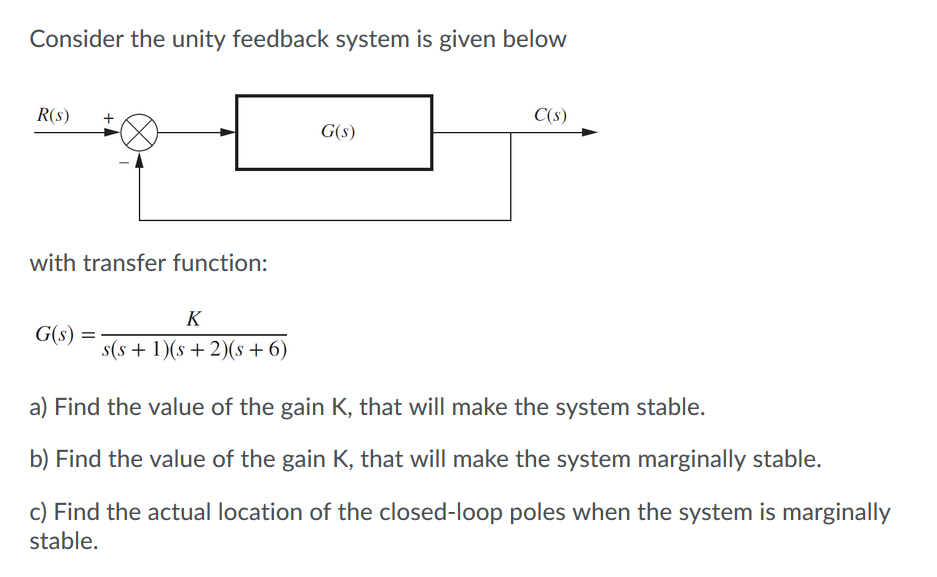Consider the unity feedback system is given below R(S) C(s) G() with transfer function: G(s) = K s(s + 1)(s + 2)(8 + 6) a) Find the value of the gain K, that will make the system stable. b) Find the value of the gain K, that will make the system marginally stable. c) Find the actual location of the closed-loop poles when the system is marginally stable.

• ### Consider the unity feedback system is given below Ris) Cs) G(8) with transfer function: -K(s +1)...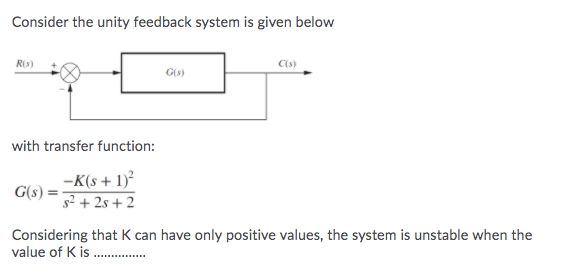Consider the unity feedback system is given below Ris) Cs) G(8) with transfer function: -K(s +1) G(S) 52 + 25 + 2 Considering that can have only positive values, the system is unstable when the value of K is ........